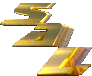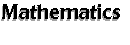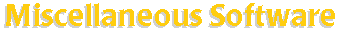[an error occurred while processing this directive] The following software do not belong to any of the other subsections in the Mathematics section. Search SAL: Miscellaneous Software   (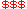Commercial,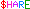Shareware,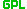GPL) Calculator: Acalc-- a console-based calculator capable of adding/subtracting/multiplying/dividing. ARIBAS-- an interactive interpreter for big integer and multi-precision floating point arithmetic. bc-- calculator with arbitrary precision numeric processing language. GNUBC PROGRAMS -- a set of programs for bc. Calc-- arbitrary precision calculator. CALC -- calculator program for doing arbitrary precision integer arithmetic. Calculator-- simple command line calculator. CCalc-- formula parsing library & command-line calculator. CMAT -- an exact arithmetic matrix calculator program. crw-- an easy to learn, easy to use, command line oriented calculator.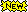Cxc-- a console calculator that works like a TI-83. equate-- simple command line calculator with precision output. gdcalc-- a GTK+-based Reverse Polish Notation calculator. Genius Calculator-- an arbitrary precision calculator for Gnome project. gexpr-- a shell calculator which is an extension of expr(1). Gnorponocal-- full-featured keyboard based RPN calculator. GNU Calc-- an advanced calculator that runs as part of the GNU Emacs environment. GRPN-- a graphical reverse polish notation calculator. GXCalc-- a programmable calculator with GTK+ interface for X. hp67-- a programmable scientific calculator emulating the HP-67. Isaac -- scientific calculator and programming language. JAVA-CALC -- a RPN type scientific calculator written in JavaScript. Java based calculator -- a simple calculator in Java applet. JavaCalc -- a simple Java Calculator written in swing. Lcalc-- a simple 4-operation calculator for Linux.L Math PRocessor-- an extension of FSF tool expr. Math-- intended to speed up tasks normally done with calculators or by hand.mmcalc-- a console-based, modular scientific calculator. Motif Calculator -- X11/Motif calculator with scientific and programming functions. MPC -- a multi-precision algebraic calculator. MoCalc -- a simple calculator written entirely in Tcl/Tk.Nickle -- a desk calculator language with powerful programming and scripting capabilities. QuickCalc-- an interactive calculator equivalent to a TI-30ish.Rascal-- a platform-independent and advanced scientific modular calculator. rcalc-- a fast and light symbolic calculator for GNOME. rpc-- a fast full-screen console-based RPN calculator. SNAC-- a simple numeric algebraic calculator for GNOME. THX-1138 -- an approximation to electronic paper which evaluates expressions, plots functions. Xforms Calculator-- a programmable scientific GUI calculator for X based on XForms. ycalc -- an X-window calculator which is heavily influenced by TI-59. Function Plotter/Mathematical Visualization: 3-D Function Builder -- a Java applet for plotting surface in 3 dimensions. Fnord -- mathematical visualization with functional and collection oriented language. fofx-- function grapher for character-cell terminals. GNOME Plotter-- graphing calculator that can display equations given in Fortran. GRAPE -- a package for mathematical visualization. GtkGraph-- an application designed to replace a conventional graphing calculator. gyapp-- a mathematical function plotting program with simulation. Function Plotter -- Java applet for polar and cartesian plots for math function. jot -- an easy-to-use f(x) function plotter supporting multiple plots. geg-- a GTK+ based simple equation grapher. Grapher-3D -- Viewing 3D surfaces plot of two variable functions. KNOT-- a graphical tool for knot theory/topology. Java Microscope -- produces vector field and performs simulations. kmplot-- mathematical function plotter for KDE. Kplot3d-- a tool for plotting 3D functions of two variables. ksciplot-- a function plotter for the KDE project. mathplot-- interactive function grapher for equations and inequations. MGrapher -- a program draws up to two math expressions. PGPA-- small graphing application for a function of one variable and many parameters. Possum-- a tool for manipulation and visualisation of partially ordered sets (posets) and domains. RICHplot -- a Java applet program to plot trigonometric functions. Spirograph -- a Java applet for creating/viewing spirograph. surf-- a tool to visualize algebraic curves and algebraic surfaces. Surface Plotter -- a Java applet plotting surface of a user supplied two variable function. Xphased -- a simple X-windows phase plane plotter. Others: Albert -- research tool to assist the study of nonassociative algebra. AMPL-- a modeling language for mathematical programming. anton-- an arithmetical game. ASCEND-- an OO mathematical modeling environment and a mathematical modeling language. AUTO -- continuation and bifurcation software for ODE. Cheng-Pleijel Point of a Quadrangle -- a Java applet to play with Cheng-Pleijel point. Chaos analysis software -- a suite of programs for chaos analysis. CinnaMoney-- is a financial class library to manage mathematics with money. CirclePack -- creation, display, manipulation, and storage of circle packings. DISCRETA -- construction of t-designs with prescribed group of automorphisms. DYNAMICAL DATA DETERMINISM DETECTOR -- a program allows time-series prediction. E-- a purely equational theorem prover for clausal logic. ehrhart -- polynomials and parametric vertices program. GENREG -- computation/construction of regular graphs. gTuring-- a simple turing machine simulator. Surface Evolver -- modeling liquid surfaces shaped by various forces. Fudgit -- data manipulation, fitting, spline. G Arith-- an arithmetic test/game for X11. Ginkgo-- a math package/graphing program. IMPS -- an interactive mathematical proof system. Knotscape -- provides convenient access to tables of knots. LEDA -- a library of efficient datatypes and algorithms. LINK -- a software system for discrete mathematics. LOLA -- a software system in location planning. LUCY -- a Clifford algebra approach to Spinor calculus. magnum-- a C++ library of fast factorisation algorithms for univariate polynomials. Magnus -- a software to explore infinite groups and carry out experiments with them.MathPlanner-- a mathematical design tool. Mathutils-- math utilities for stdin/stdout script programming. Mtk-- handy software for evaluation, differentiation, integration, root finding, graphing etc. MVPoly -- a multi-variable polynomial algebra package in Perl. Mx -- a combination of a matrix algebra interpreter and a numerical optimizer. nnsort -- generating the Delaunay tesselation or convex hull in 2D or 3D. Nuprl -- a mathematical proof development system. Oorange -- a software framework for experimental mathematics. OR-Objects -- a library of Java classes for developing Operation Research applications. Otter -- system designed to prove theorems stated in first-order logic with equality. PolyLib -- operates on objects made up of unions of polyhedra of any dimension. prime -- a prime number generator. Qhull -- a general dimension code for computing convex hulls, Delaunay triangulations, etc. Sheafhom -- a set of programs for homological algebra and algebraic topology. Symmetrica -- a collection of routines for group theory, etc. TMath -- a Tcl interface to Matlab and Mathematica. ZEN-- toolbox for fast computations in finite extensions of finite rings. Also Check Out: ACL2-- programming language to model computer system and prove properties. Cinderella -- an interactive geometry software written in Java. Dr Genius-- geometry exploration and numeric intuitive user system. Gnuplot -- visualize mathematical functions and data. SaGA-- Matlab toolbox for geometrical modeling and spatial data analysis. UnitCalc -- a calculator which does all arithmetic with the numbers and the units.Number Theory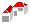SAL Home   |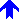Mathematics